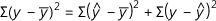# Statistics Symbols in Alphabetical Order

Share on

Statistics Basics > Statistics Symbols in Alphabetical Order

## Probability and Statistics Symbols

I’ve put these statistics symbols into quasi-alphabetical order, so that they are easy to find. When I say quasi-alphabetical, I mean that I have ordered them according to what they look like. For example, the Greek letter Beta (β) looks like the letter b, so you’ll find it in the b section. Same for the letter mu(μ), which looks like the letter u.cIf a statistics symbol doesn’t look like a letter, for example the “Greater than” symbol, >, you’ll find it at the very bottom under “miscellaneous.” Most items are clickable, taking you to an article that explains the statistics symbols in more depth.

## Statistics Symbols A to Zα: significance level (type I error).
b or b0: y intercept.
b1: slope of a line (used in regression).
β: probability of a Type II error.
1-β: statistical power.
BD or BPD: binomial distribution.
CI: confidence interval.
CLT: Central Limit Theorem.
d: difference between paired data.
df: degrees of freedom.
DPD: discrete probability distribution.
E = margin of error.
f = frequency (i.e. how often something happens).
f/n = relative frequency.
HT = hypothesis test.
Ho = null hypothesis.
H1 or Ha: alternative hypothesis.
IQR = interquartile range.
m = slope of a line.
M: median.
n: sample size or number of trials in a binomial experiment.
N: population size.
ND: normal distribution.
σ: standard deviation.
σx̅: standard error of the mean.
σp̂: standard error of the proportion.
p: p-value, or probability of success in a binomial experiment, or population proportion.
ρ: correlation coefficient for a population.
: sample proportion.
P(A): probability of event A.
P(AC) or P(not A): the probability that A doesn’t happen.
P(B|A): the probability that event B occurs, given that event A occurs.
Pk: kth percentile. For example, P90 = 90th percentile.
q: probability of failure in a binomial or geometric distribution.
Q1: first quartile.
Q3: third quartile.
r: correlation coefficient of a sample.
R2: coefficient of determination.
s: standard deviation of a sample.
s.d or SD: standard deviation.
SEM: standard error of the mean.
SEP: standard error of the proportion.
t: t-score.
μ mean.
ν: degrees of freedom.
X: a variable.
Χ2: chi-square.
x: one data value.
: mean of a sample.
z: z-score.

Miscellaneous Statistics Symbols
~ has the distribution of (source).
= equal to.
almost equal to.
> greater than.
< less than.
not equal to.
less than or equal to.
greater than or equal to.
Σ Summation.

## References

Everitt, B. S.; Skrondal, A. (2010), The Cambridge Dictionary of Statistics, Cambridge University Press.
Gonick, L. (1993). The Cartoon Guide to Statistics. HarperPerennial.
Lindstrom, D. (2010). Schaum’s Easy Outline of Statistics, Second Edition (Schaum’s Easy Outlines) 2nd Edition. McGraw-Hill Education

CITE THIS AS:
Stephanie Glen. "Statistics Symbols in Alphabetical Order" From StatisticsHowTo.com: Elementary Statistics for the rest of us! https://www.statisticshowto.com/statistics-symbols/
---------------------------------------------------------------------------Need help with a homework or test question? With Chegg Study, you can get step-by-step solutions to your questions from an expert in the field. Your first 30 minutes with a Chegg tutor is free!